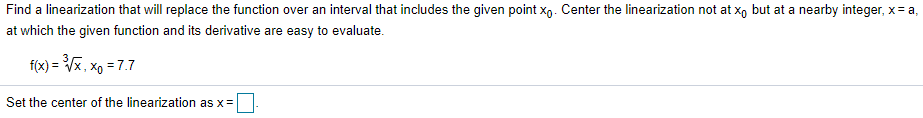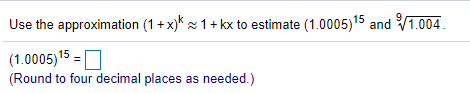### Create an Account

Home / Questions / Find a linearization that will replace the function over an interval that includes the giv...

# Find a linearization that will replace the function over an interval that includes the given point Xo. Center the linearization not atxo but at a nearby integer, x= a, at which the given function and

Find a linearization that will replace the function over an interval that includes the given point Xo. Center the linearization not atxo but at a nearby integer, x= a, at which the given function and its derivative are easy to evaluate. f(x) = XX. Xo = 7.7 Set the center of the linearization as x=
Use the approximation (1 + x)* 1 + kx to estimate (1.0005) 15 and 1.004. (1.0005)15 = (Round to four decimal places as needed.)Apr 28 2021 View more View LessSubscribe To Get Solution